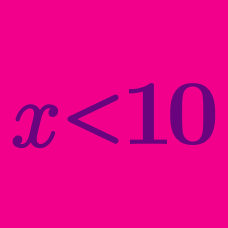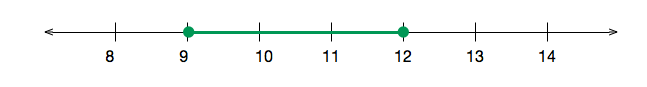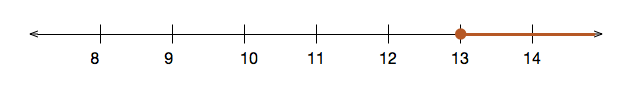Algebra

# Finding Solutions to Linear InequalitiesWhich inequality is represented in the above number line?

True or false?

$18 \leq 18$

Which of the following values of $x$ does not satisfy $12\leq3x-3<34?$

If $x$ is an integer that satisfies $-4 what is the sum of all possible values of $x?$Which inequality is represented in the above number line?

×